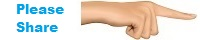Classroom Pronunciation Reductions Grammar Videos Conversation Reading Activities Listening Study Idioms Slang Acronyms Surveys Tests Alphabet Alphabet 4 Kids Vocabulary Travel America Drive America About Contact Copyright Resources Site MapSubtraction Zero and Positive Integers
 Flashcards 4 Kids In this lesson you will use flashcards to improve your English. Read the flashcards description, instructions, information, and do the flashcards.
 Flashcards 4 Kids - Subtraction Zero and Positive Integers
 Flashcards 4 Kids - Subtraction Zero and Positive Integers Description This lesson has mathematics flashcards with descriptions in English. Flashcards 4 Kids - Subtraction Zero and Positive Integers Instructions Press the PLAY button to begin the flashcard presentation each slide shows for 3 seconds Press the FORWARD button to advance one slide ahead with no time limit Press the BACK button to advance one slide back with no time limit Use a dictionary if you cannot understand a word
 Flashcards 4 Kids - Subtraction Zero and Positive Integers Information This set of Fun Easy English flashcards contains the following calculations along with their descriptions: 1 - 1 = 0 2 - 1 = 1 2 - 2 = 0 3 - 1 = 2 3 - 2 = 1 3 - 3 = 0 4 - 1 = 3 4 - 2 = 2 4 - 3 = 1 4 - 4 = 0 5 - 1 = 4 5 - 2 = 3 5 - 3 = 2 5 - 4 = 1 5 - 5 = 0 6 - 1 = 5 6 - 2 = 4 6 - 3 = 3 6 - 4 = 2 6 - 5 = 1 6 - 6 = 0 7 - 1 = 6 7 - 2 = 5 7 - 3 = 4 7 - 4 = 3 7 - 5 = 2 7 - 6 = 1 7 - 7 = 0 8 - 1 = 7 8 - 2 = 6 8 - 3 = 5 8 - 4 = 4 8 - 5 = 3 8 - 6 = 2 8 - 7 = 1 8 - 8 = 0 9 - 1 = 8 9 - 2 = 7 9 - 3 = 6 9 - 4 = 5 9 - 5 = 4 9 - 6 = 3 9 - 7 = 2 9 - 8 = 1 9 - 9 = 0 10 - 1 = 9 10 - 2 = 8 10 - 3 = 7 10 - 4 = 6 10 - 5 = 5 10 - 6 = 4 10 - 7 = 3 10 - 8 = 2 10 - 9 = 1 10 - 10 = 0 Please post a comment below if these flashcards are no longer working. Add Flashcards 4 Kids - Subtraction Zero and Positive Integers to YOUR page, blog, website Simply cut and paste the following: Send Flashcards 4 Kids - Subtraction Zero and Positive Integers to YOUR friends Simply cut and paste the following: https://docs.google.com/presentation/pub?id=14oDJ34hJRS-yEyUseZ-48oYq-vqUIVr-z-Wx6KMHdfc&start=false&loop=false&delayms=3000